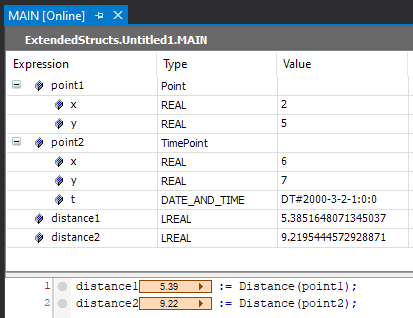I was wondering if overloading functions in TwinCAT was possible, but I found out that this is not the case. I did however realize that overloading is possible using a few work-arounds. In this article I’ll show how to mimic this behavior with extended structs.

Overloading a function means that you can call a function with an identical name, but it can have a different number of arguments. Also the data type of the arguments and/or the return type can differ. Some programming languages support more overloading types then others, as you can see here in this overview.

An example of overloading in C# would be the following. The function `Rectangle` can be called using a either a single `int` argument (making it a square), or it can be called using two `float` arguments. Furthermore the single argument function returns an `int`, whereas the two argument one returns a `float`.

``````public static class Area
{
public static int Rectangle(int a)
{
return a * a;
}

public static float Rectangle(float a, float b)
{
return a * b;
}
}
``````

Many methods in C# are overloaded. See for example the `Sum` method with an impressive 20 overloads!

Jakob Sagatowski showed in his post “The wonders of any” that you can call a single function with different data types. In order to make this work he uses `ANY`.

You can also use extended structs to have a single function which accepts multiple structs which use the same base struct. For example we have a `Point` struct with a x and a y coordinate.

``````TYPE Point :
STRUCT
x : REAL;
y : REAL;
END_STRUCT
END_TYPE
``````

And an extension of this struct which can also hold the time and a date.

``````TYPE TimePoint EXTENDS Point :
STRUCT
t : DT;
END_STRUCT
END_TYPE
``````

Then we make a function which calculates the distance of this point with respect to the origin at (0, 0).

``````FUNCTION Length : LREAL
VAR_INPUT
point : Point;
END_VAR

Length := SQRT(EXPT(point.x, 2) + EXPT(point.y, 2));
``````

Finally we call the method on two different points. One which is of type `Point` and the second which is of type `TimePoint`.

``````PROGRAM MAIN
VAR
point1 : Point := (x:=2, y:=5);
point2 : TimePoint := (x:=6, y:=7, t:=DT#2000-03-02-1:0);
length1 : LREAL;
length2 : LREAL;
END_VAR

length1 := Length(point1);
length2 := Length(point2);
``````

When we run this program we can see that it calculated the length for both points.Using this trick you can prevent having to write/test the same function multiple times. I couldn’t find any mention of this possibility on InfoSys, so I just tried it out. I was a bit surprised and relieved that it worked!

Discuss: r/plc & r/TwinCAT

Found a mistake or a typo? Suggest a change!